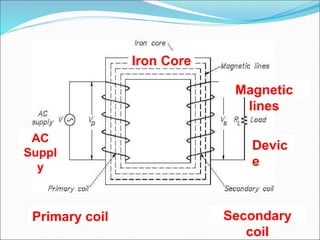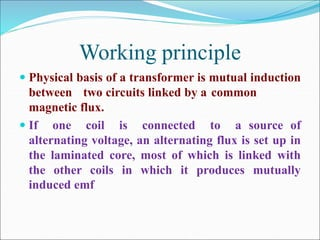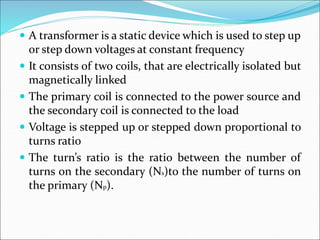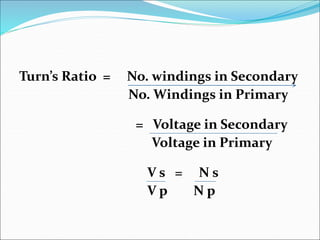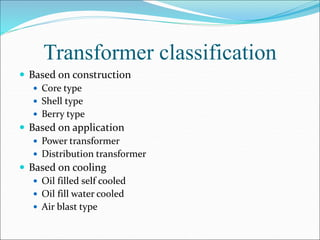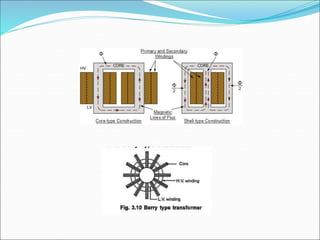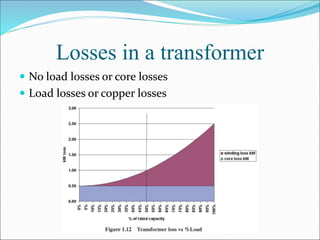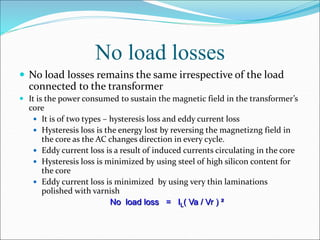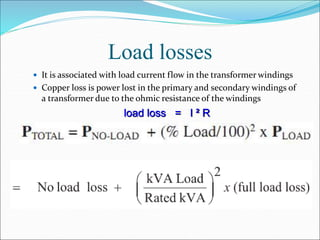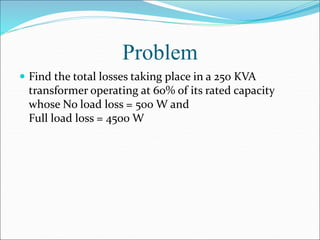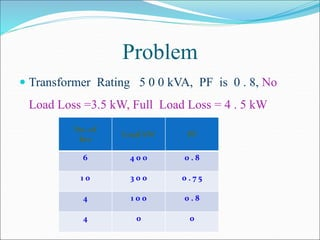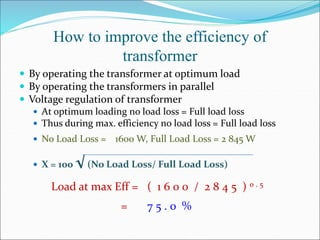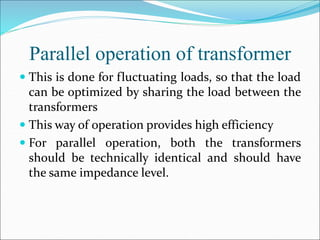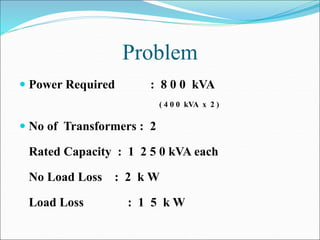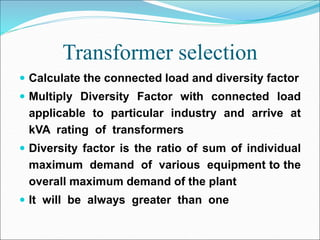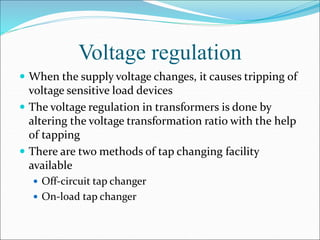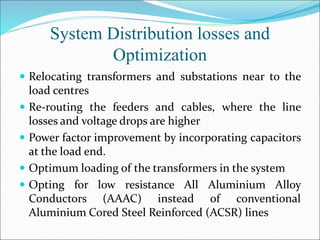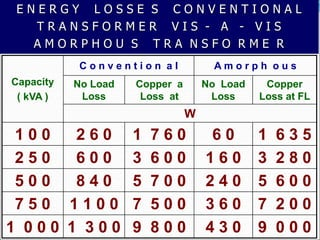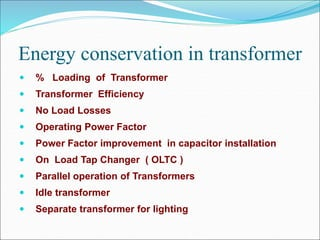1 de 20

### presentation_transformers_1489551826_236516.ppt

1. Iron Core Magnetic lines Devic e Secondary coil Primary coil AC Suppl y
2. Working principle  Physical basis of a transformer is mutual induction between two circuits linked by a common magnetic flux.  If one coil is connected to a source of alternating voltage, an alternating flux is set up in the laminated core, most of which is linked with the other coils in which it produces mutually induced emf
3.  A transformer is a static device which is used to step up or step down voltages at constant frequency  It consists of two coils, that are electrically isolated but magnetically linked  The primary coil is connected to the power source and the secondary coil is connected to the load  Voltage is stepped up or stepped down proportional to turns ratio  The turn’s ratio is the ratio between the number of turns on the secondary (Ns)to the number of turns on the primary (Np).
4. Turn’s Ratio = No. windings in Secondary No. Windings in Primary = Voltage in Secondary Voltage in Primary V s = N s V p N p
5. Transformer classification  Based on construction  Core type  Shell type  Berry type  Based on application  Power transformer  Distribution transformer  Based on cooling  Oil filled self cooled  Oil fill water cooled  Air blast type
6. Losses in a transformer  No load losses or core losses  Load losses or copper losses
7. No load losses  No load losses remains the same irrespective of the load connected to the transformer  It is the power consumed to sustain the magnetic field in the transformer’s core  It is of two types – hysteresis loss and eddy current loss  Hysteresis loss is the energy lost by reversing the magnetizng field in the core as the AC changes direction in every cycle.  Eddy current loss is a result of induced currents circulating in the core  Hysteresis loss is minimized by using steel of high silicon content for the core  Eddy current loss is minimized by using very thin laminations polished with varnish No load loss = IL( Va / Vr ) ²
8. Load losses  It is associated with load current flow in the transformer windings  Copper loss is power lost in the primary and secondary windings of a transformer due to the ohmic resistance of the windings load loss = I ² R
9. Problem  Find the total losses taking place in a 250 KVA transformer operating at 60% of its rated capacity whose No load loss = 500 W and Full load loss = 4500 W
10. Problem  Transformer Rating 5 0 0 kVA, PF is 0 . 8, No Load Loss =3.5 kW, Full Load Loss = 4 . 5 kW No. of hrs Load kW PF 6 4 0 0 0 . 8 1 0 3 0 0 0 . 7 5 4 1 0 0 0 . 8 4 0 0